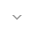# Interpolator

`public class Interpolator`
`extends Object`

 java.lang.Object ↳ android.graphics.Interpolator

## Summary

### Nested classes

`枚举` `Interpolator.Result`

### Public constructors

` Interpolator(int valueCount) `
` Interpolator(int valueCount, int frameCount) `

### Public methods

` final int` ` getKeyFrameCount() `
` final int` ` getValueCount() `
` void` ` reset(int valueCount) `

` void` ` reset(int valueCount, int frameCount) `

` void` ` setKeyFrame(int index, int msec, float[] values, float[] blend) `

` void` ` setKeyFrame(int index, int msec, float[] values) `

` void` ` setRepeatMirror(float repeatCount, boolean mirror) `

` Interpolator.Result` ` timeToValues(int msec, float[] values) `

` Interpolator.Result` ` timeToValues(float[] values) `

### Protected methods

` void` ` finalize() `

### Inherited methodsFrom class ` java.lang.Object `

## Public constructors

### Interpolator

`Interpolator (int valueCount)`

Parameters
`valueCount` `int`

### Interpolator

```Interpolator (int valueCount,
int frameCount)```

Parameters
`valueCount` `int`
`frameCount` `int`

## Public methods

### getKeyFrameCount

`int getKeyFrameCount ()`

Returns
`int`

### getValueCount

`int getValueCount ()`

Returns
`int`

### reset

`void reset (int valueCount)`

Parameters
`valueCount` `int`

### reset

```void reset (int valueCount,
int frameCount)```

Parameters
`valueCount` `int`
`frameCount` `int`

### setKeyFrame

```void setKeyFrame (int index,
int msec,
float[] values,
float[] blend)```

Parameters
`index` `int`: The index of the key frame to assign
`msec` `int`: The time (in mililiseconds) for this key frame. Based on the SystemClock.uptimeMillis() clock
`values` `float`: Array of values associated with theis key frame
`blend` `float`: (may be null) Optional array of 4 blend values

### setKeyFrame

```void setKeyFrame (int index,
int msec,
float[] values)```

Parameters
`index` `int`: The index of the key frame to assign
`msec` `int`: The time (in mililiseconds) for this key frame. Based on the SystemClock.uptimeMillis() clock
`values` `float`: Array of values associated with theis key frame

### setRepeatMirror

```void setRepeatMirror (float repeatCount,
boolean mirror)```

Parameters
`repeatCount` `float`
`mirror` `boolean`

### timeToValues

```Interpolator.Result timeToValues (int msec,
float[] values)```

Parameters
`msec` `int`: The time (in milliseconds) used to sample into the Interpolator. Based on the SystemClock.uptimeMillis() clock
`values` `float`: Where to write the computed values (may be NULL).
Returns
`Interpolator.Result` how the values were computed (even if values == null)

### timeToValues

`Interpolator.Result timeToValues (float[] values)`

Parameters
`values` `float`
Returns
`Interpolator.Result`

## Protected methods

### finalize

`void finalize ()`

`finalize`方法`Object`执行特殊的操作; 它只是正常返回。 `Object`子类可能会覆盖此定义。

Java编程语言不保证哪个线程将为任何给定的对象调用`finalize`方法。 但是，保证调用finalize的线程在调用finalize时不会保留任何用户可见的同步锁。 如果finalize方法引发未捕获的异常，则忽略该异常，并终止该对象的终止。

`finalize`方法抛出的任何异常 `finalize`导致此对象的终止被暂停，但在其他情况下会被忽略。

Throws
`Throwable`